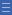# Activity: Magic Vs

Activity: Magic Vs

Place each of the numbers from 1 to 5 in a V shape so that the two arms of the V have the same total sum.

## Find the activity on NRICH

Go to http://nrich.maths.org/6274 (link will open in a new window)

## More questions

What happens if we use the numbers a, a + 1, a + 2, a + 3, a + 4? What are the possible sums of each arm?

What is the largest number on each arm if we use the numbers a, a + 1, a + 2,… in a V of arm length n? What are the possible sums for each arm?

Could you use a set of numbers that does not differ by 1? If so, what must be special about the numbers?

Could you use a set of integers so that the sum of each arm is 0?

## Shine a light on the Proficiencies

Asking “How many different possibilities are there?” shows that mathematical problems often have more than answer. An important question to ask is how we know we have found all the possible answers, which highlights the importance of reasoning.

Algebraic formulation requires students to look at the structure of the problem rather than at specific numbers. Structure is fundamental to being able to reason mathematically.

The V diagram can be used to introduce the concept of an arithmetic sequence, while asking for the sum of each arm enables students to generate the formula for the sum of an arithmetic series.

A useful practice to encourage problem solving is to reverse the question. In this case asking students to try to create a V shape with a sum of 0 brings in both negative numbers and requires student to give careful thought to which number needs to be placed at the apex of the V.

Yes

Yes

Name Class Section PriorityDownload Magic Vs (blank worksheet) File 1